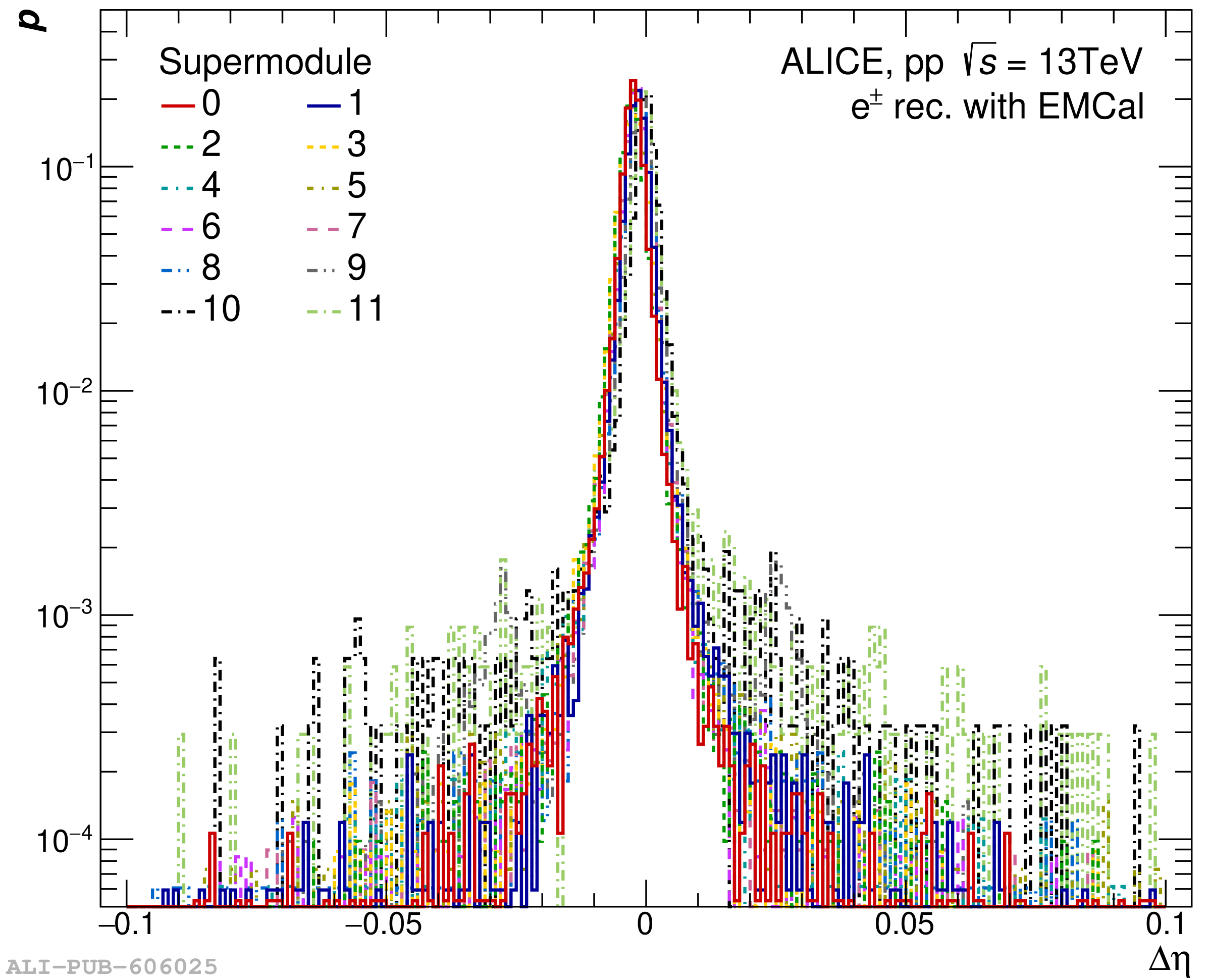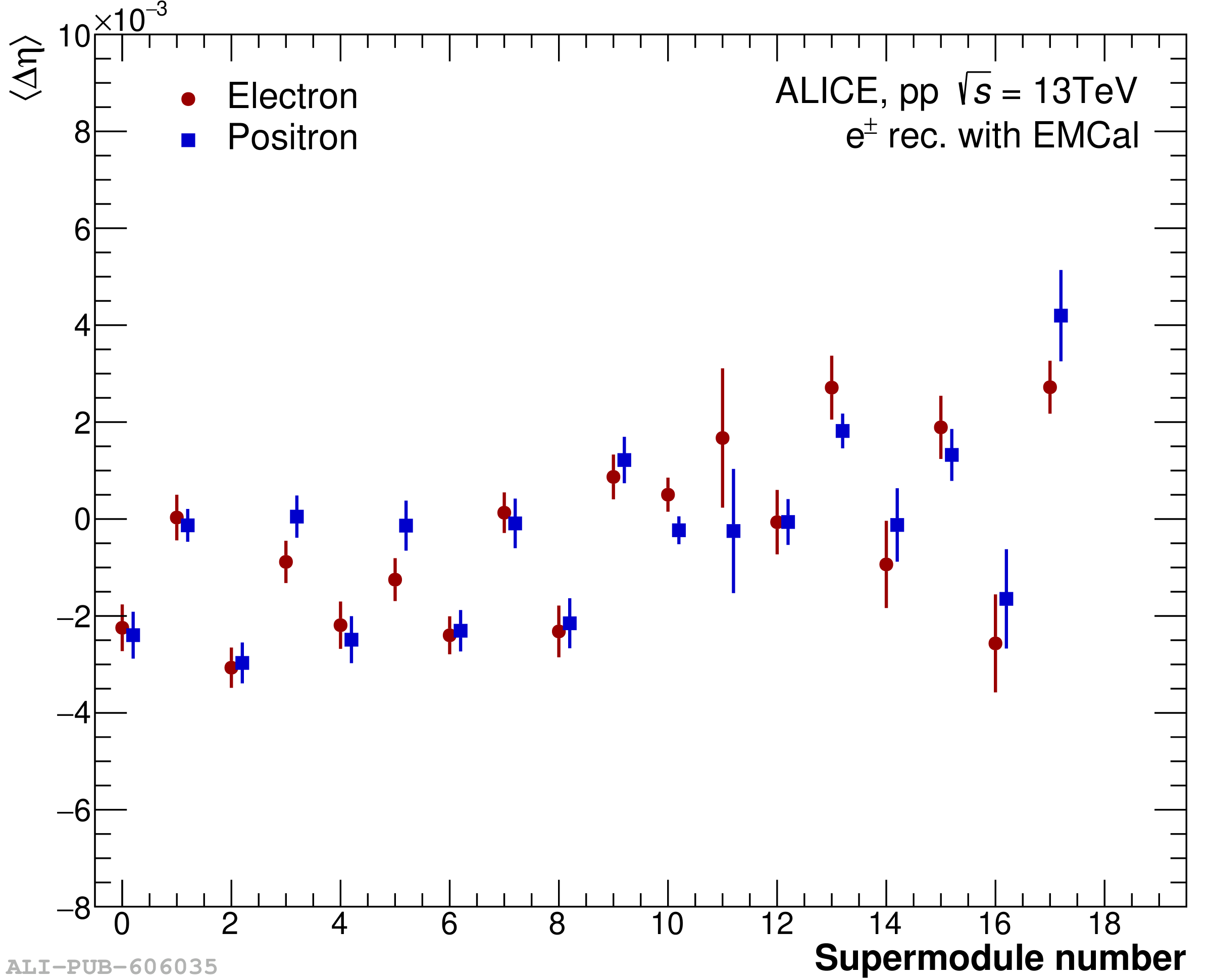# Figure 43

 The probability distribution of the position of electron tracks propagated to the \gls{EMCal} \glspl{SM} surface and their associated cluster in $\eta$-direction (left) and $\varphi$-direction (right). The distributions are normalized by their integral. Mean differences between the position in $\eta$ (left) and $\varphi$ (right) of clusters and electron tracks propagated to the \gls{EMCal} / \gls{DCal} as function of the \gls{SM} number. The colors represent electrons (red) and positrons (blue).